# Hydrazine (N2H4) lewis dot structure, molecular geometry, polarity, hybridization

Home  > Chemistry Article > N2H4 lewis structure and its molecular geometryHydrazine is highly toxic composed of two nitrogen and four hydrogens having the chemical formula N2H4. It has an odor similar to ammonia and appears colorless. It is also known as nitrogen hydride or diazane. It is corrosive to tissue and used in various rocket fuels.

In this article, we will discuss N2H4 lewis structure, molecular geometry, hybridization, bond angle, polarity, etc.

Hydrazine is toxic by inhalation and by skin absorption. Long-term exposure to hydrazine can cause burning, nausea, shortness of breath, dizziness, and many more health-related problems.

Properties of Hydrazine

• It is miscible in water.
• It appears as a colorless and oily liquid.
• It has a boiling point of 114 °C and a melting point of 2 °C.
• It is a strong base and has a conjugate acid(Hydrazinium).
• The dipole moment of N2H4 is 1.85 D.
 Name of Molecule Hydrazine Chemical formula N2H4 Molecular geometry of N2H4 Trigonal pyramidal Electron geometry of N2H4 Tetrahedral Hybridization Sp³ Bond angle 107° – 109.5º Total Valence electron in N2H4 lewis structure 14
Page Contents

## How to draw N2H4 lewis structure?

N2H4 lewis structure is made up of two nitrogen and four hydrogens having two lone pairs on the nitrogen atoms(one lone pair on each nitrogen) and contain a total of 10 shared electrons.

There are three types of bonds present in the N2H4 lewis structure, one N-N, and two H-N-H.

Let’s start the construction of the N2H4 lewis dot structure step by step-

So, here we go!

## Follow some steps for drawing the Lewis dot structure of N2H4

1. Count total valence electron in N2H4

As we know, lewis’s structure is a representation of the valence electron in a molecule. So, in the first step, we have to count how many valence electrons are available for N2H4.

I assume that you are definitely know how to find the valence electron of an atom. The valence electron of an atom is equal to the periodic group number of that atom.

So, nitrogen belongs to the 15th periodic group, and hydrogen to the 1st group. Therefore, the valence electron for nitrogen is 5 and for hydrogen, it is 1.

⇒ Total number of the valence electrons in nitrogen = 5

⇒ Total number of the valence electrons in hydrogen = 1

∴ Total number of valence electron available for the N2H4 lewis structure = 5(2) + 1(4) = 14 valence electrons         [∴two nitrogen and four hydrogen]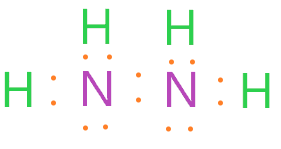2. Find the least electronegative atom and placed it at center

Now it’s time to find the central atom of the N2H4 molecule. It doesn’t matter which atom is more or less electronegative, if hydrogen atoms are there in a molecule then it always goes outside in the lewis diagram.

Because hydrogen only needs two-electron or one single bond to complete the outer shell. So, for N2H4, put away hydrogen outside and nitrogen as a central atom in the lewis diagram.3. Connect outer atoms to central atom with a single bond

In this step, we need to connect every outer atom(hydrogen) to the central atom(nitrogen) with the help of a single bond. And make sure you must connect both nitrogens with a single bond also.Now count the total number of valence electrons we used till now in the above structure. A single bond contains two-electron and as we see in the above structure, 5 single bonds are used, hence we used 10 valence electrons till now.

We had 14 total valence electrons available for drawing the N2H4 lewis structure and from them, we used 10 valence electrons.

∴ (14 – 10) = 4 valence electrons

So, we are left with 4 valence electrons more.

4. Place remaining valence electrons starting from outer atom first

Now we have to place the remaining valence electron around the outer atom first, in order to complete their octet. Lewis’s structure is all about the octet rule.

Octet rule said that each elements tend to bond in such a way that each atom has eight electrons in its valence shell

Always remember, hydrogen is an exception to the octet rule as it needs only two electrons to complete the outer shell. As hydrogen has only one shell and in one shell, there can be only two electrons.

So, as you see in the 3rd step structure, all hydrogen atoms completing their octet as they already sharing two electrons with the help of a single bond.

Now head over to the next step-

5. Complete central atom octet and make covalent bond if necessary

As hydrogen atom already completed their octet, we have to look at the central atom(nitrogen) in order to complete its octet. Nitrogen needs 8 electrons in its outer shell to gain stability, hence achieving octet.

If you look at the structure in the 3rd step, each nitrogen has three single bonds around it. So, each nitrogen already sharing 6 valence electrons(3 single bonds).

In order to complete the octet, we need two more electrons for each nitrogen. We have already 4 leftover valence electrons in our account.

So, put two and two on each nitrogen. Hurry up!### N2H4 lewis dot structure

Yes, we completed the octet of both atoms(nitrogen and hydrogen) and also used all available valence electrons.

I think we completed the lewis dot structure of N2H4? No, we need one more step to verify the stability of the above structure with the help of the formal charge concept.

6. Check the stability with the help of a formal charge concept

A formal charge is the charge assigned to an atom in a molecule, assuming that electrons in all chemical bonds are shared equally between atoms.

The structure with the formal charge close to zero or zero is the best and stable lewis structure.

To calculate the formal charge on an atom. Use the formula given below-

⇒ Formal charge = (valence electrons – lone pair electrons –  1/2shared pair electrons)

We will calculate the formal charge on the individual atoms of the N2H4 lewis structure.

For nitrogen atom:

⇒ Valence electrons of nitrogen = 5

⇒ Lone pair electrons on nitrogen = 2

⇒ Shared pair electrons(3 single bond) = 6

(5 – 2 – 6/2) = 0 formal charge on the nitrogen atom

For hydrogen atom

⇒ Valence electrons of hydrogen = 1

⇒ Lone pair on hydrogen = 0

⇒ Shared pair electrons(one single bond) = 2

(1 – 0 – 2/2) = o formal charge on the hydrogen atom

Hence, the overall formal charge in the N2H4 lewis structure is zero.

Therefore, we got our best lewis diagram.

## What is the molecular geometry of N2H4?

“Molecular geometry is the three-dimensional arrangement of atoms in a molecule”. The molecular geometry of N2H4 is trigonal pyramidal as each nitrogen(left side or right side) is attached to two hydrogen atoms and there is one lone pair on it.

The lone pair electron present on nitrogen and shared pair electrons(around nitrogen) will repel each other according to the VSEPR theory. As a result, they will be pushed apart giving the trigonal pyramidal geometry on each nitrogen side.

Since both nitrogen sides are symmetrical in the N2H4 structure, hence there shape will also be the same.

This is the only overview of the N2H4 molecular geometry. Now we will learn, How to determine the shape of N2H4 through its lewis diagram?

We will use the AXN method to determine the geometry. Generally, AXN is the representing of electron pairs(Bond pairs + Lone pairs) around a central atom, and after that by applying the VSEPR theory, we will predict the shape of the geometry of the molecule.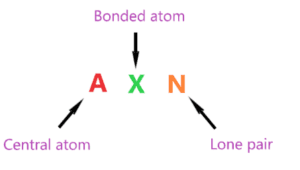That’s how the AXN notation follows as shown in the above picture.

Now we have to find the molecular geometry of N2H4 by using this method.

AXN notation for N2H4 molecule:

• A represents the central atom, so as per the N2H4 lewis structure, nitrogen is the central atom. A = Nitrogen
• X represents the bonded atoms, as we know, nitrogen is making three bonds(two with hydrogen and one with nitrogen also). Therefore, X = 3
• N represents the lone pair, nitrogen atom has one lone pair on it. Hence, N = 1

So, the AXN notation for the N2H4 molecule becomes AX3N1.

As per the VSEPR theory and its chart, if a molecule central atom is attached with three bonded atoms and has one lone pair then the molecular geometry of that molecule is trigonal pyramidal.

Hence, the molecular shape or geometry for N2H4 is trigonal pyramidal.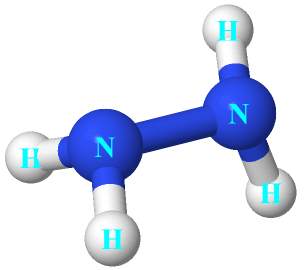### N2H4 molecular geometry

As you see in the molecular shape of N2H4, on the left side, nitrogen is attached to the two hydrogen atoms and both are below of plane of rotation and on the right side, one hydrogen is above and one is below in the plane.

The electron geometry for N2H4 is tetrahedral.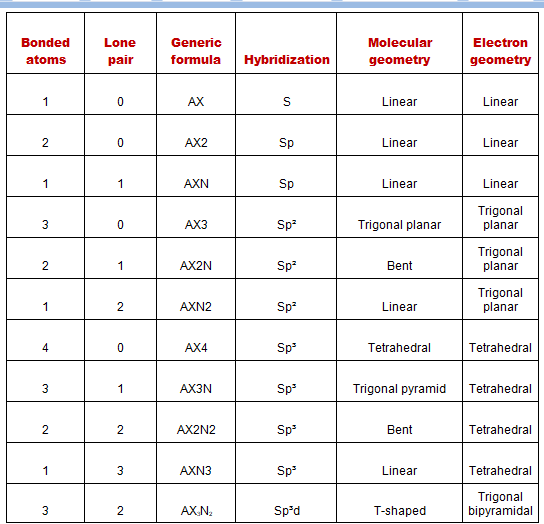## Hybridization of N2H4

To find the hybridization of an atom, we have to first determine its hybridization number.

Hybridization number is the addition of a total number of bonded atoms around a central atom and the lone pair present on it.

∴ Hybridization number of N2H4 = (Number of bonded atoms attached to nitrogen + Lone pair on nitrogen)

According to the N2H4 lewis dot structure, we have three bonded atoms attached to the nitrogen and one lone pair present on it.

∴ Hybridization number of N2H4 = (3 + 1) = 4

 Hybridization number Hybridization 1 S 2 Sp 3 Sp² 4 Sp³ 5 Sp³d 6 Sp³d²

So, for a hybridization number of four, we get the Sp3 hybridization on each nitrogen atom in the N2H4 molecule.

## The bond angle of N2H4

“A bond angle is the geometrical angle between two adjacent bonds”.

The bond angle of N2H4 is subtended by H-N-H and N-N-H will be between 107° – 109°. Normally, atoms that have Sp3 hybridization hold a bond angle of 109.5°. Since one lone pair is present on the nitrogen atom in N2H4, lower the bond angle to some extent.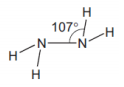## Hydrazine polarity: is N2H4 polar or nonpolar

We know, there is one lone pair on each nitrogen in the N2H4 molecule, both nitrogens is Sp3 hybridized. One hybrid of each orbital forms an N-N bond. But the bond N-N is non-polar because of the same electronegativity and the N-H bond is polar because of the slight difference between the electronegativity of nitrogen and hydrogen.

As you see the molecular geometry of N2H4, on the left side and right side, there is the total number of four N-H bonds present. Three hydrogens are below their respective nitrogen and one is above.

Also, the presence of lone pair on each nitrogen distorted the shape of the molecule since the lone pair tries to repel with bonded pair. So, there is no point that they will cancel the dipole moment generated along with the bond.

As both sides in the N2H4 structure seem symmetrical to different planes i.e. left side symmetric to the vertical plane(both hydrogen below) and the right side symmetric to the horizontal plane(one hydrogen is below and one is above).So, the resultant of four N-H bond moments and two lone electron pairs leads to the dipole moment of 1.85 D. hence, N2H4 is a polar molecule.

## FAQ

### How many shared pair electrons and lone pair electrons the N2H4 lewis structure contains?

Shared pair electrons are also called the bonded pair electrons as they make the covalent between two atoms and share the electrons. Lone pair electrons are unshared electrons means they don’t take part in chemical bonding.

Shared pair electrons in N2H4 molecule = a total of 10 shared pair electrons(5 single bonds) are present in N2H4 molecule.

Lone pair electrons in N2H4 molecule = Both nitrogen central atom contains two lone pair. So, lone pair of electrons in N2H4 equals, 2 ×(2) = 4 unshared electrons.

### Why is there no double bond in the N2H4 lewis dot structure?

As you closely see the N2H4 lewis structure, hydrogen can occupy only two electrons in its outer shell, which means hydrogen can share only two electrons. So, there is no point we can use a double bond with hydrogen since a double bond contains a total of 4 electrons.

Now let’s talk about the N-N bond, each nitrogen has three single bonds and one lone pair. If we convert the lone pair into a covalent bond then nitrogen shared four bonds(two single and one double bond).

But the problem is if a double bond is present in the N2H4 dot structure, then it becomes unstable. As nitrogen atom will get some formal charge.

“Lewis structure is most stable when the formal charge is close to zero”.

That’s why there is no need to make any double or triple bond as we already got our best and stable N2H4 lewis structure with zero formal charges.

## Summary

• The total valence electron available for the N2H4 lewis structure is 14.
• The hybridization of each nitrogen in the N2H4 molecule is Sp3.
• N2H4 is polar in nature and dipole moment of 1.85 D.
• The formal charge on nitrogen in N2H4 is zero.
• Total 2 lone pairs and 5 bonded pairs present in N2H4 lewis dot structure.
• The molecular geometry of N2H4 is trigonal pyramidal and its electron geometry is tetrahedral.
##### Subscribe to Blog via Email

Join 2 other subscribers

Share it...